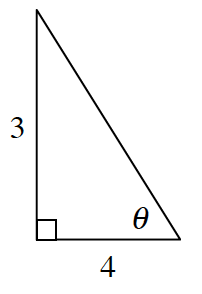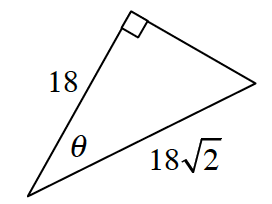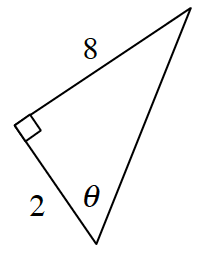### Home > GC > Chapter 4 > Lesson 4.2.5 > Problem4-91

4-91.

Based on the measurements provided for each triangle below, decide if the angle $θ$ must be more than, less than, or equal to $45º$. Assume the diagram is not drawn to scale. Show how you know.

1.Think about what the slope ratio must be for the angle to be $45º$.

1.Use the Pythagorean Theorem to solve for the missing leg. What does this length tell you about the angle's measure?

1.Use the same method as in part (a).

More than $45º$